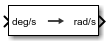Angular Velocity Conversion

Convert from angular velocity units to desired angular velocity units

• Library:
• Aerospace Blockset / Utilities / Unit Conversions

•Description

The Angular Velocity Conversion block computes the conversion factor from specified input angular velocity units to specified output angular velocity units and applies the conversion factor to the input signal.

The Angular Velocity Conversion block port labels change based on the input and output units selected from the Initial unit and the Final unit lists.

Ports

Input

expand all

Angular acceleration, specified as a scalar, in initial angular acceleration units.

Dependencies

The input port label depends on the Initial unit setting.

Data Types: double

Output

expand all

Angular acceleration, returned as a scalar, in final angular acceleration units.

Dependencies

The output port label depends on the Final unit setting.

Data Types: double

Parameters

expand all

Input units, specified as:

 deg/s Degrees per second rad/s Radians per second rpm Revolutions per minute

Dependencies

The input port label depends on the Initial unit setting.

Programmatic Use

 Block Parameter: IU Type: character vector Values: 'deg/s' | 'rad/s' | 'rpm/s' Default: 'deg/s'

Output units, specified as:

 deg/s Degrees per second rad/s Radians per second rpm Revolutions per minute

Dependencies

The output port label depends on the Final unit setting.

Programmatic Use

 Block Parameter: OU Type: character vector Values: 'deg/s' | 'rad/s' | 'rpm/s' Default: 'deg/s'

Extended Capabilities

C/C++ Code GenerationGenerate C and C++ code using Simulink® Coder™.

Introduced before R2006a

Aerospace Blockset DocumentationGet trial now